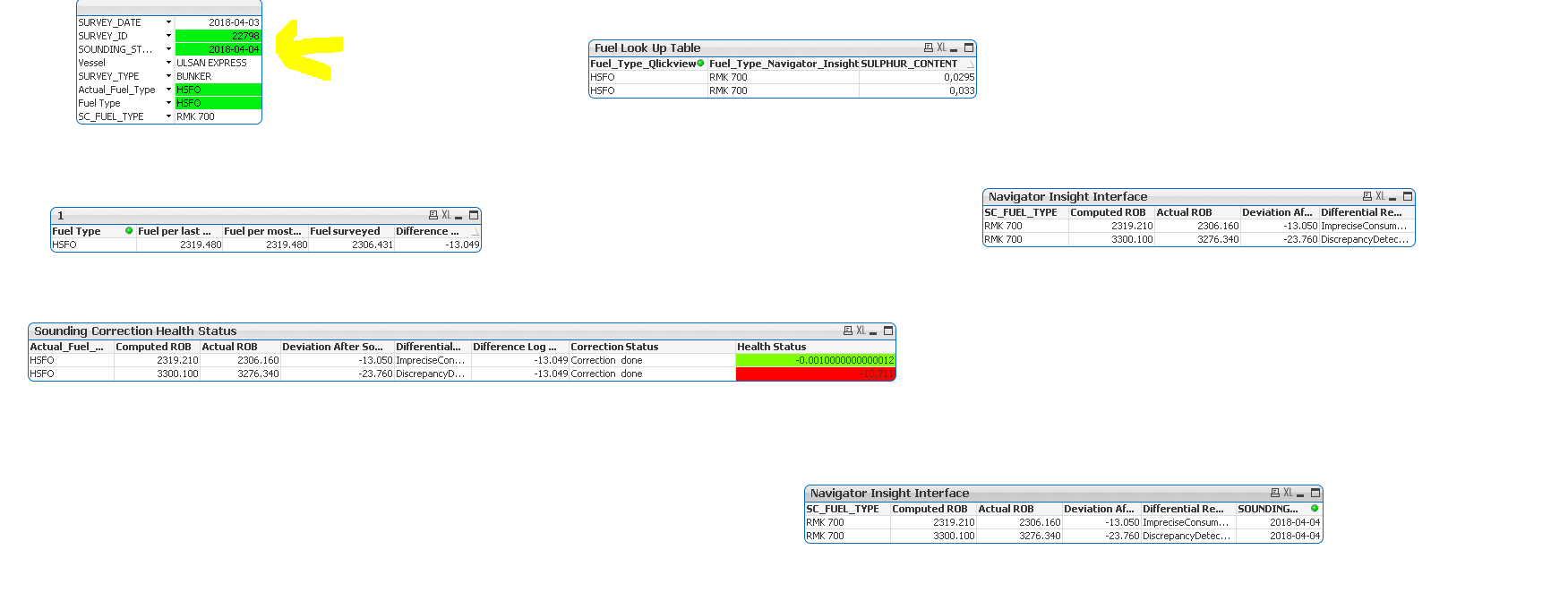# New to QlikView

Discussion board where members can get started with QlikView.

Contributor III

## How can I fetch data in a given interval of time?

I have a logic to fetch data: I need to fetch data for all those records on sounding date  which start after a survey starts and ends within 7 days of the survey start date.When we get no data or if there are no such records then an error is generated like correction not done

If([SOUNDING_START_DATE] >= [SURVEY_START_DATE] and [SOUNDING_START_DATE] < date(max([SURVEY_START_DATE] ))+ 7, SURVEY_ID, 0)

I also tried using interval match but some of the dates and records which are not matching,seems to disappear from the report hence I wanted a simple approach to work with chart expressions.

Any help is accepted

5 Replies
Valued Contributor II

## Re: How can I fetch data in a given interval of time?

Esteemed Contributor

## Re: How can I fetch data in a given interval of time?

like mentioned above, set analysis with range of dates can be the solution in the front end.. if you're stuck after reading those threads, you can attach sample data here to help you

Highlighted
Contributor III

## Re: How can I fetch data in a given interval of time?I am stuck here actually.I want that whenever I give a survey date, it will automatically pickup the sounding date within a range of the survey date and 7 days within it..If there are no such matches then it will throw an error and i can count the survey ids for which we dont have any sounding data...But at first I would like to select the date:  If([SOUNDING_START_DATE] >= [SURVEY_START_DATE] and [SOUNDING_START_DATE] < date(max([SURVEY_START_DATE] ))+ 7, SURVEY_ID, 0)

or something related to it..so that if the survey  start date is 3.04.2018 then then the sounding date it should be pick will be in the range 7 days from 3.04.2018--

Insimple words for a survey start date at 3.04.2018, it will pickup the sounding dates within 3.04.18 to 10.04.18

Honored Contributor

## Re: How can I fetch data in a given interval of time?

May be like this:

If([SOUNDING_START_DATE] <= [SURVEY_START_DATE] + 7, SURVEY_ID,0) as Flag

Or

If([SOUNDING_START_DATE] <= max([SURVEY_START_DATE]) + 7, SURVEY_ID,0) as Flag

Or

If([SOUNDING_START_DATE] <= max([SURVEY_START_DATE]) + 7, Count(SURVEY_ID),0) as Flag

Contributor III

## Re: How can I fetch data in a given interval of time?

But how can i put such condition in a multiple list box